Have questions? Need Help?
757.723.6531 | 800.950.2468

# Teledyne Hastings Instruments Blog

One of the goals of these blog postings is to give readers knowledge about vacuum and mass flow technology. The Society of Vacuum Coaters has established a foundation (SVCF) with a similar goal. Dr. Don McClure (Acuity Consulting & Training) has created “The Vacuum Wizard Video”. Dr. McClure worked at both IBM & 3M and has been teaching for over 20 years about vacuum coating onto flexible substrates.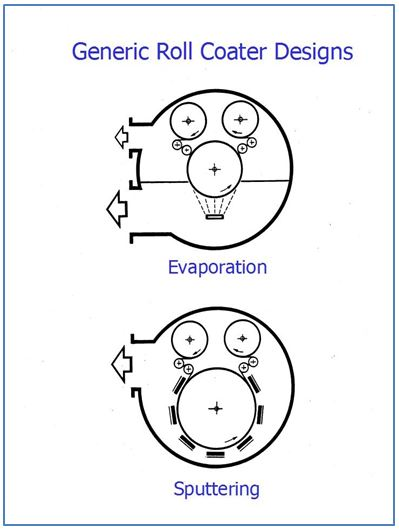As stated on the SVCF website, “The Vacuum Wizard Video brings to life the fundamentals of vacuum and vacuum coating technology through an informal and thought provoking presentation using non-technical jargon and filled with live demonstrations.

The Vacuum Wizard Video seeks to raise awareness of students and educators about the fascinating world of vacuum and vacuum coating technology. The only prerequisite is a curiosity about this amazing technology.

The Vacuum Wizard Video can be a useful training tool in the corporate world for personnel who require a basic understanding of vacuum technology. Sales representatives, customer service personnel, field service and maintenance technicians, lab technicians, and engineers with no vacuum technology background, can all benefit from the Vacuum Wizard Video.”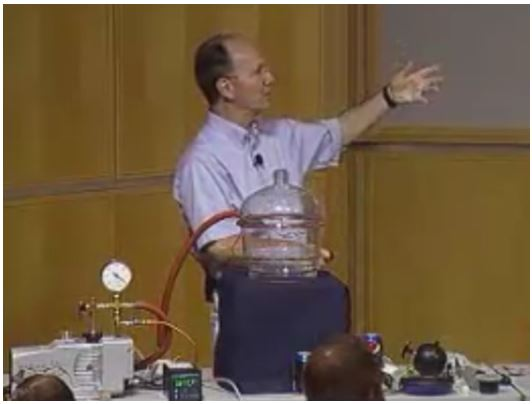(Check out the Teledyne Hastings’ Vacuum Model 2002 Vacuum Gauge on the table)  Click the button below to request an evaluation sample of the 2002 Vacuum GaugeYou can get more information about the SVC Foundation and the video series by visiting:

http://svcfoundation.org

Click to see a sample of the Vacuum Wizard Video

Tags: Mass Flow, vacuum gauges

Teledyne Hastings is proud to offer our Mass Flow Converter app. We have created a version for iPhone, iPad, and Droid. We have also created a web-based version that you can find at www.massflowconverter.com

In this blog article, we will discuss the motivation to build the app, how it works, and how it can be used.

The first question you might be asking is: Why do we need an app to convert from one set of mass flow units to another? For instance, if you want to convert from inches to centimeters, you would just multiply by 2.54. But, converting between mass flow units is not always that straight forward. So we have developed a tool that makes it easy.

We are going to look at some examples, but first let’s review what we mean by mass flow. When we think about mass flow, it can be helpful to think in terms of the flow of individual molecules. So while flow meters are often specified by units like sccm (standard cubic centimeters per minute) or scfm (standard cubic feet per minute), the mass flow rate is ultimately about the number of molecules (n) moving through a given cross sectional area per unit time (see figure below).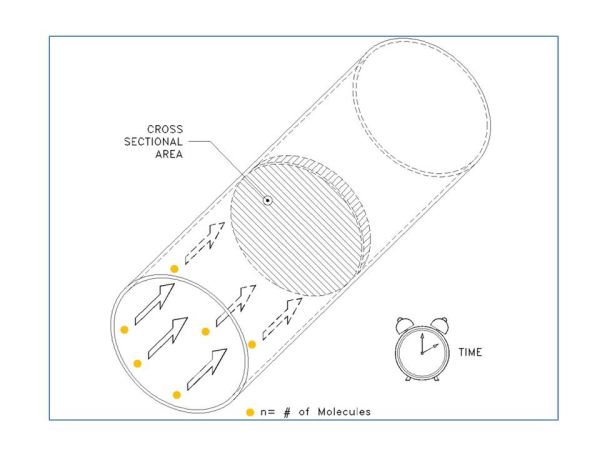So as our first example, let’s take a look at the conversion of 10,000 sccm (10,000 cm3/min) to a molecular flow rate. First, we need to ask, “How many molecules are in 10,000 sccm?” In the figure below, we show a container that is 10,000 cm3 in volume. Now, before we can calculate the number of gas molecules in a volume, we must know the pressure and temperature of the gas. We can use the ideal gas law:

n = (P * V) / R*T where n is the number of molecules, P is the pressure, V is the Volume, R is the Universal Gas Constant and T is the Temperature.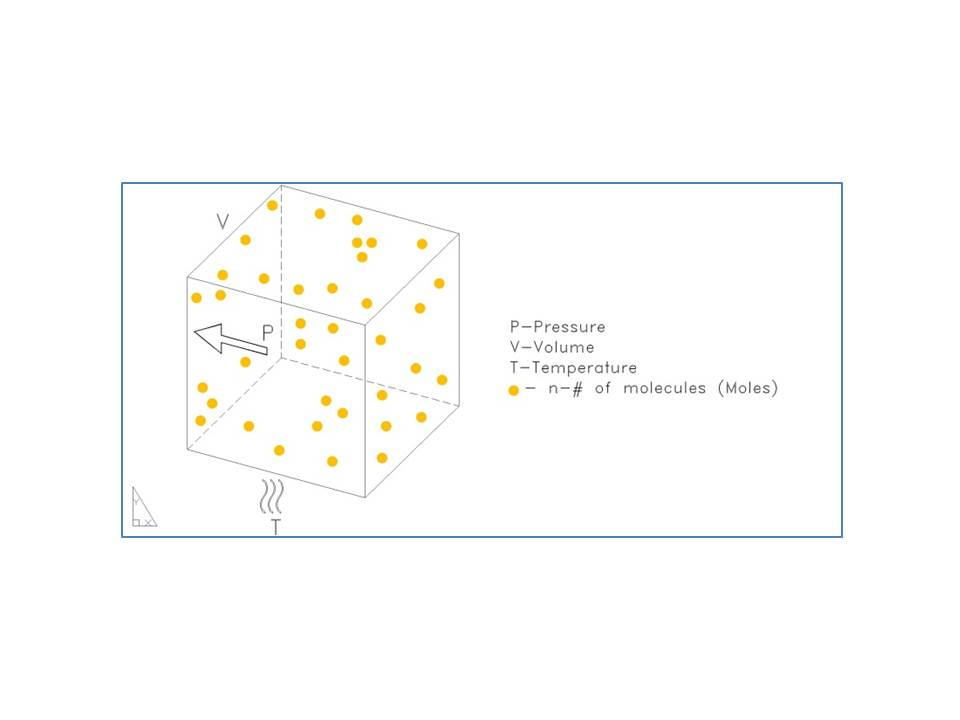Now we need to select some given pressure and temperature so that we can calculate the number of molecules – these are called the reference conditions or the STP (Standard Temperature and Pressure). In many cases, 0°C and 760 Torr are used for the STP. But this is not always the case. So it is always very important to specify the reference conditions (STP) any time you use a standardized mass flow unit like sccm, slm, scfh, etc (any mass flow unit that starts with “s” is going to need the reference conditions or STP specified). In our example, we are going to use STP of 0°C & 760 Torr.

OK, so here we go:     n = (1 atm) * (10,000 cc) / (82.053 cc * atm / K * mole) * (273 K)

Note that we have used a value of R in terms of pressure in Atmosphere (760 Torr = 1 atm), and Temperature in Kelvin (0°C = 273K).

n = 0.45 mole

In other words, a flow rate of 10,000 sccm (0C, 760 Torr) is the same as a molecular flow rate of 0.45 Mole / minute.

OK that is the hard way. It’s much easier to use the mass flow converter app. In the example shown above, we would dial sccm on the left and Mole/Min on the right. Then to select the reference conditions, we use the menu in the center. See Fig. 3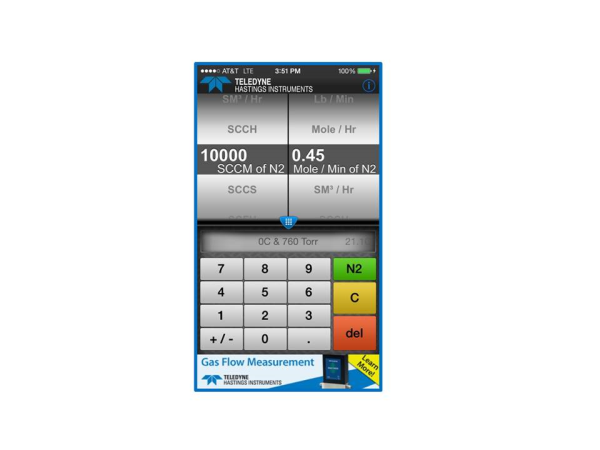If you are like me, you will start to play with the App. And soon you will notice that the user can change the gas using the pull down menu at the top. But notice that in the case of our first example (converting from standardized mass flow units to molecular flow units), that the gas selection has no effect on the conversion.  This is because the standardized flow units (e.g. sccm, slm, scfh, etc.) are actually molar flow units based on reference conditions (STP) and the ideal gas law.

So, why do we allow the user to select gas? In the case of units like gm/sec, Kg/hr, or lb / min, we are going to need to know the gas so that we can calculate the mass. Let’s take a look at the case of converting from slm to grams/second. We will use as our same example of 10,000 slm (0°C & 760 Torr) and we will use methane (CH4) as our gas.

We showed earlier that 10,000 sccm is a molecular flow rate of 0.45 Mole / Min.  And since 1 slm = 1000 sccm, it is easy to see that 10,000 slm = 450 Mol/min. And since we know that our unit of choice (gm/sec) is in terms of seconds, let’s go ahead and convert our time units now:

10,000 slm = (450 Mole / Min) * (1 Min / 60 sec) = 7.5 Mole/ sec.

Now we need to know how much mass there is in a Mole of methane. Google is very nice for getting this number – just type, “Molecular weight of methane” and here is the result: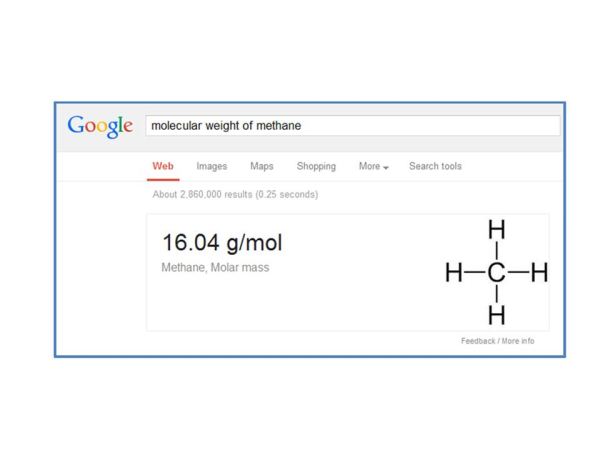By the way, Google will do this for almost all gases. So now we can finish our conversion and we get:

7.5 Mol / sec * (16.04 g/mol) = 120 g/sec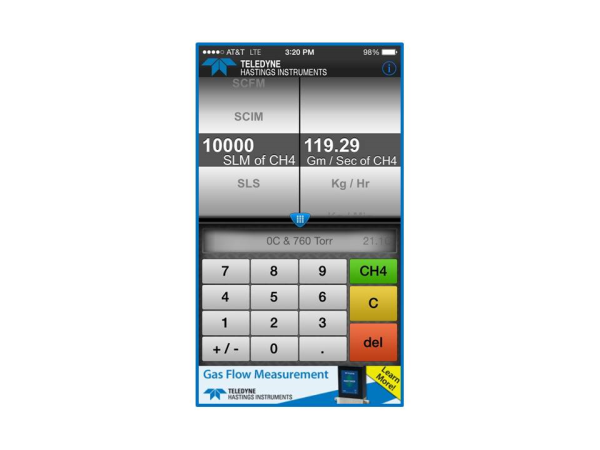The mass flow converter app and website www.massflowconverter.com takes all the work out of these conversions and we hope that you will find this tool helpful. If you have any questions about mass flow meters and controllers, Application Engineers at Teledyne Hastings are always happy to help.